##Electromagnetic induction : 6 Dear Readers, JEE Advanced Physics Syllabus can be referred by the IIT aspirants to get a detailed list of all topics that are important in cracking the entrance examination. JEE Advanced syllabus for Physics has been designed in such a way that it offers very practical and application-based learning to further make it easier for students to understand every concept or topic by correlating it with day-to-day experiences. In comparison to the other two subjects, the syllabus of JEE Advanced for physics is developed in such a way so as to test the deep understanding and application of concepts.. .question { margin-bottom: 20px; border-bottom: 1px solid #ddd; } .ques { font-size: 15px; font-weight: bold; line-height: 150%; } .q-options { padding: 0 !important; list-style: none; margin: 15px 0 !important; } .opt { font-size: 14px; font-weight: normal; } .answer { color: blue; display: none; padding-bottom: 15px; line-height: 150%; } .opt-right.active { background: #a3ff91; font-size: 19px; line-height: 150%; border: 1px solid ##a3ff91; -webkit-border-radius: 5px; -moz-border-radius: 5px; border-radius: 5px; margin: 10px 0; } .mcq-info { margin: 20px 0 40px; color: #060553; line-height: 150%; } .timer { overflow: hidden; margin: 20px 0; background: #f7f7f7; padding: 10px; border: 1px solid #eee; border-radius: 3px; } .start-btn, .time-btn, .stop-btn, #reset-start { border: 1px solid #777; -o-border-radius: 2px; -ms-border-radius: 2px; -webkit-border-radius: 2px; border-radius: 2px; color: #fff; padding: 7px 15px; width: auto; height: auto; line-height: 150%; background: #0061ae; cursor: pointer; margin-right: 10px; } #reset-start { background: #0e2230; color: #fff; border-color: #0e2230; display: none; } .start-btn:hover, .time-btn:hover, .stop-btn:hover { background: #0e2230; color: #fff; border-color: #0e2230; } #reset-start:hover { background: #0061ae; color: #fff; border-color: #0061ae; } @media screen and (max-width: 767px) { .ques, .answer, .mcq-info, .opt { font-size: 13px; } } Q1.  Switch S of the circuit shown in Fig is closed at t=0If e denotes the induced e.m.f. in L and i the current flowing through the circuit at time t, then which of the following graphs correctly represents the variation of e with i?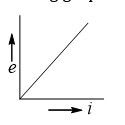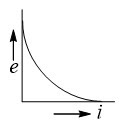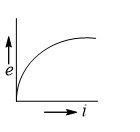Solution b Q2.A uniform magnetic field of induction B fills a cylindrical volume of radius R. A rod AB of length 2l is placed as shown in figure. If B is changing at the rate dB/dt, the emf that is produced by the changing magnetic field and that acts between the ends of the rod is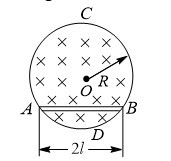dB/dt l√(R2-l2 )  dB/dt l√(R2+l2 )  1/2 dB/dt l√(R2-l2 )  1/2 dB/dt l√(R2+l2 ) Solution a Q3.  The frequency of oscillation of current in the inductance is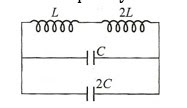1/(3√LC)  1/(6π√LC)  1/√LC  1/(2π√LC) Solution b Q4.  A uniform but time-varying magnetic field B(t) exists in a circular region of radius a and is directed into the plane of the paper, as shown. The magnitude of the induced electric filed at point P at a distance r from the centre of the circular region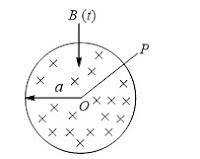Is zero  Decreases as 1/r  Increases as r  Decreases as 1/r2 Solution b Q5.A wire of fixed length is wound on a solenoid of length l and radius r. Its self-inductance is found to be L. Now, if the same wire is wound on a solenoid of length l/2 and radius r/2, then the self-inductance will be  2L  4L  L  8L Solution  a Q6. 6. An e.m.f of 15 V is applied in a circuit containing 5 H inductance and 10 Ω resistance. The ratio of the currents at time t=∞ and t=1 s is  e(1/2)/(e(1/2)-1)  e2/(e2-1))  1-e-1  e-1 Solution b Q7.Calculate the inductance of a unit length of a double tape line as shown in fig, if the tapes are separated by a distance h which is considerably less than their width b(μ0 h)/b  (μ0 h)/2b  (2μ0 h)/b  (√2 μ0 h)/bt Solution a Q8. The figure shows certain wire segments joined together to form a coplanar loop. The loop is placed in a perpendicular magnetic field in the direction going into the plane of the figure. The magnitude of the field increases with time. I1 and I2 are the currents in the segments ab and cd . Then,I1>I2  TI2>I1  I1is in the direction ba and I2is in the direction cd  I1is in the direction ab and I2is in the direction dc Solution d Q9.In fig, the mutual inductance of a coil and a very long straight wire is M, the coil has resistance R and self-inductance L. The current in the wire varies according to the law i=at, where a is a constant and t is the time, the time dependence of current in the coil is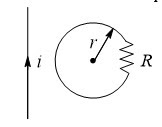M/aR  MaRe-Rt/L  M/R e-tR/L  Ma/R(1-e-tR/L) Solution d Q10. The current generator i_g, shown in figure, sends a constant current i through the circuit. The wire ab has a length l and mass m slide on the smooth, horizontal rails connected to i_g. The entire system lies in a vertical magnetic field B. The velocity of the wire as a function of time is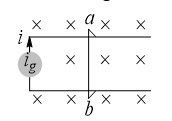ilBt/m  ilBt/2m  2ilBt/m  ilBt/3m Solution a //Answers array of your Quiz var answers = ["b", "a", "b", "b", "a", "b", "a", "d", "d", "a"]; var tot = answers.length; function getCheckedValue(radioName) { var radios = document.getElementsByName(radioName); //console.log(radios) for (var y = 0; y < radios.length; y++) { if (radios[y].checked) return radios[y].value; } } function getScore() { var score = 0; for (var i = 0; i < tot; i++) { var answerVal = getCheckedValue("question" + i); //console.log(answerVal, answers[i]) if (typeof answerVal != undefined && answerVal == answers[i]) score += 1; } return score; } function returnScore() { alert("Your score is" + getScore() + "/" + tot); } var q, m, s; function quizCount() { if (!q) { console.log("mobile2"); (m = 1), (s = 0); } if (q) { clearTimeout(q); } (m = 10), (s = 0); \$("#timer").val(m + ":" + s); s = s - 1; console.log(s); q = setTimeout("counter()", 1000); if (s < 1) { m = m - 1; console.log(m); s = 59; } } function counter() { console.log(m); \$("#timer").val(m + ":" + s); s = s - 1; console.log(s); q = setTimeout("counter()", 1000); if (s < 1) { m = m - 1; console.log(m); s = 59; } if (m < 0) { quizStop(); } } function quizStop() { clearTimeout(q); q = null; m = null; s = null; document.getElementById("timer").value = "Quiz Finished"; alert("Your score is " + getScore() + "/" + tot); \$(".answer").slideDown(); \$(".opt-right").addClass("active"); \$(".start-btn, #reset").hide(); \$("#reset-start").show(); } function quizReset() { clearTimeout(q); document.getElementById("timer").reset(); } \$(document).ready(function () { // var a = \$('.question .q-options li .rad').length; \$(".submit").click(function () { returnScore(); \$(".opt-right").addClass("active"); \$(".answer").slideDown(); }); \$("#reset").click(function () { quizReset(); }); \$("#reset-start").click(function () { \$(".opt-right").removeClass("active"); \$(".answer").slideUp(); \$(".start-btn, #reset").show(); \$("#reset-start").hide(); \$(".rad").prop("checked", false); }); });

JEE Advanced Physics Syllabus can be referred by the IIT aspirants to get a detailed list of all topics that are important in cracking the entrance examination. JEE Advanced syllabus for Physics has been designed in such a way that it offers very practical and application-based learning to further make it easier for students to understand every concept or topic by correlating it with day-to-day experiences. In comparison to the other two subjects, the syllabus of JEE Advanced for physics is developed in such a way so as to test the deep understanding and application of concepts..

Q1.  Switch S of the circuit shown in Fig is closed at t=0 If e denotes the induced e.m.f. in L and i the current flowing through the circuit at time t, then which of the following graphs correctly represents the variation of e with i?
Solution
b

Q2.A uniform magnetic field of induction B fills a cylindrical volume of radius R. A rod AB of length 2l is placed as shown in figure. If B is changing at the rate dB/dt, the emf that is produced by the changing magnetic field and that acts between the ends of the rod is
•   dB/dt l√(R2-l2 )
•  dB/dt l√(R2+l2 )
•  1/2 dB/dt l√(R2-l2 )
•  1/2 dB/dt l√(R2+l2 )
Solution
a

•  1/(3√LC)
•  1/(6π√LC)
•  1/√LC
•  1/(2π√LC)
Solution
b

Q4.  A uniform but time-varying magnetic field B(t) exists in a circular region of radius a and is directed into the plane of the paper, as shown. The magnitude of the induced electric filed at point P at a distance r from the centre of the circular region
•  Is zero
•  Decreases as 1/r
•  Increases as r
•  Decreases as 1/r2
Solution
b

Q5.A wire of fixed length is wound on a solenoid of length l and radius r. Its self-inductance is found to be L. Now, if the same wire is wound on a solenoid of length l/2 and radius r/2, then the self-inductance will be
•  2L
•  4L
•  L
•  8L
Solution
a

Q6. 6. An e.m.f of 15 V is applied in a circuit containing 5 H inductance and 10 Ω resistance. The ratio of the currents at time t=∞ and t=1 s is
•  e(1/2)/(e(1/2)-1)
•  e2/(e2-1))
•  1-e-1
•  e-1
Solution
b

Q7.Calculate the inductance of a unit length of a double tape line as shown in fig, if the tapes are separated by a distance h which is considerably less than their width b
•  0 h)/b
•  0 h)/2b
•  (2μ0 h)/b
•  (√2 μ0 h)/bt
Solution
a

Q8. The figure shows certain wire segments joined together to form a coplanar loop. The loop is placed in a perpendicular magnetic field in the direction going into the plane of the figure. The magnitude of the field increases with time. I1 and I2 are the currents in the segments ab and cd . Then,
•   I1>I2
•  TI2>I1
•  I1is in the direction ba and I2is in the direction cd
•  I1is in the direction ab and I2is in the direction dc
Solution
d

Q9.In fig, the mutual inductance of a coil and a very long straight wire is M, the coil has resistance R and self-inductance L. The current in the wire varies according to the law i=at, where a is a constant and t is the time, the time dependence of current in the coil is
•  M/aR
•  MaRe-Rt/L
•  M/R e-tR/L
•  Ma/R(1-e-tR/L)
Solution
d

Q10. The current generator i_g, shown in figure, sends a constant current i through the circuit. The wire ab has a length l and mass m slide on the smooth, horizontal rails connected to i_g. The entire system lies in a vertical magnetic field B. The velocity of the wire as a function of time is
•  ilBt/m
•  ilBt/2m
•  2ilBt/m
•  ilBt/3m
Solution
a#### Written by: AUTHORNAME

AUTHORDESCRIPTION## Want to know more

Please fill in the details below:

## Latest NEET Articles\$type=three\$c=3\$author=hide\$comment=hide\$rm=hide\$date=hide\$snippet=hide

Name

ltr
item
BEST NEET COACHING CENTER | BEST IIT JEE COACHING INSTITUTE | BEST NEET & IIT JEE COACHING: Electromagnetic Induction - Quiz 6
Electromagnetic Induction - Quiz 6
https://1.bp.blogspot.com/-ClFrMz19-h8/YN7qVPzO2_I/AAAAAAAAQPA/wbXGk20pGsA5IBvoANCLDdKRKdi9y-lKwCLcBGAsYHQ/s320/Quiz%2BImage%2B20.jpg
https://1.bp.blogspot.com/-ClFrMz19-h8/YN7qVPzO2_I/AAAAAAAAQPA/wbXGk20pGsA5IBvoANCLDdKRKdi9y-lKwCLcBGAsYHQ/s72-c/Quiz%2BImage%2B20.jpg
BEST NEET COACHING CENTER | BEST IIT JEE COACHING INSTITUTE | BEST NEET & IIT JEE COACHING
https://www.cleariitmedical.com/2021/07/electromagnetic-induction-quiz-6.html
https://www.cleariitmedical.com/
https://www.cleariitmedical.com/
https://www.cleariitmedical.com/2021/07/electromagnetic-induction-quiz-6.html
true
7783647550433378923
UTF-8

STAY CONNECTED